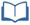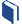# Making Deviation Standard

Estimated Time: 90 minutes

###Lesson Objectives:

• The students will describe and analyze sets of data using the mean and mean absolute deviation.
• The students will describe and analyze sets of data using the mean and standard deviation.
• The students will compare and contrast mean absolute deviation and standard deviation.

###Resources for this lesson:

Before you begin, download your Algebra II Journalfor Making Deviation Standard. You will be completing activities in the journal throughout this lesson.

Visit this section on each page to access information about key terms and other resources to help facilitate the lesson.

> Glossary> Calculator Resources> Teacher Resources: Instructional NotesKey Common Core State Standards:

S.ID.4: Use the mean and standard deviation of a data set to fit it to a normal distribution and to estimate population percentages. Recognize that there are data sets for which such a procedure is not appropriate. Use calculators, spreadsheets, and tables to estimate areas under the normal curve.

Supporting Common Core State Standards:

6.SP.5c: Giving quantitative measures of center (median and/or mean) and variability (interquartile range and/or mean absolute deviation), as well as describing any overall pattern and any striking deviations from the overall pattern with reference to the context in which the data were gathered.

S.ID.2: Use statistics appropriate to the shape of the data distribution to compare center (median, mean) and spread (interquartile range, standard deviation) of two or more different data sets.

S.ID.3: Interpret differences in shape, center, and spread in the context of the data sets, accounting for possible effects of extreme data points (outliers).

Standards for Mathematical Practices:

1. Make sense of problems and persevere in solving them.
2. Reason abstractly and quantitatively.
4. Model with mathematics.
5. Use appropriate tools strategically.

Next >